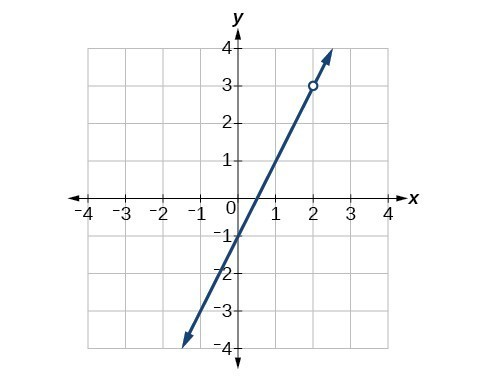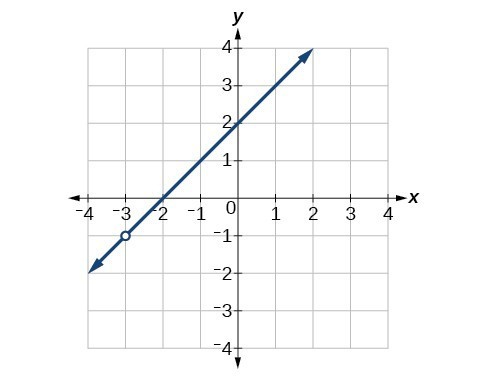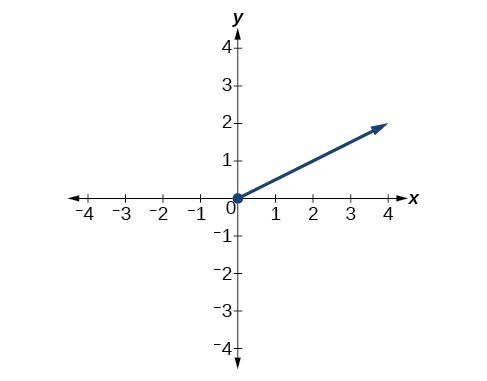## Section 9.2: Finding Limits: Properties of Limits

### Learning Outcomes

• Find the limit of a sum, a difference, and a product.
• Find the limit of a polynomial.
• Find the limit of a power or a root.
• Find the limit of a quotient.

## Finding the Limit of a Sum, a Difference, and a Product

Graphing a function or exploring a table of values to determine a limit can be cumbersome and time-consuming. When possible, it is more efficient to use the properties of limits, which is a collection of theorems for finding limits.

Knowing the properties of limits allows us to compute limits directly. We can add, subtract, multiply, and divide the limits of functions as if we were performing the operations on the functions themselves to find the limit of the result. Similarly, we can find the limit of a function raised to a power by raising the limit to that power. We can also find the limit of the root of a function by taking the root of the limit. Using these operations on limits, we can find the limits of more complex functions by finding the limits of their simpler component functions.

### A General Note: Properties of Limits

Let $a,k,A$, and $B$ represent real numbers, and $f$ and $g$ be functions, such that $\underset{x\to a}{\mathrm{lim}}f\left(x\right)=A$ and $\underset{x\to a}{\mathrm{lim}}g\left(x\right)=B$. For limits that exist and are finite, the properties of limits are summarized in the table below.

 Constant, k $\underset{x\to a}{\mathrm{lim}}k=k$ Constant times a function $\underset{x\to a}{\mathrm{lim}}\left[k\cdot f\left(x\right)\right]=k\underset{x\to a}{\mathrm{lim}}f\left(x\right)=kA$ Sum of functions $\underset{x\to a}{\mathrm{lim}}\left[f\left(x\right)+g\left(x\right)\right]=\underset{x\to a}{\mathrm{lim}}f\left(x\right)+\underset{x\to a}{\mathrm{lim}}g\left(x\right)=A+B$ Difference of functions $\underset{x\to a}{\mathrm{lim}}\left[f\left(x\right)-g\left(x\right)\right]=\underset{x\to a}{\mathrm{lim}}f\left(x\right)-\underset{x\to a}{\mathrm{lim}}g\left(x\right)=A-B$ Product of functions $\underset{x\to a}{\mathrm{lim}}\left[f\left(x\right)\cdot g\left(x\right)\right]=\underset{x\to a}{\mathrm{lim}}f\left(x\right)\cdot \underset{x\to a}{\mathrm{lim}}g\left(x\right)=A\cdot B$ Quotient of functions $\underset{x\to a}{\mathrm{lim}}\dfrac{f\left(x\right)}{g\left(x\right)}=\dfrac{\underset{x\to a}{\mathrm{lim}}f\left(x\right)}{\underset{x\to a}{\mathrm{lim}}g\left(x\right)}=\dfrac{A}{B},B\ne 0$ Function raised to an exponent $\underset{x\to a}{\mathrm{lim}}{\left[f\left(x\right)\right]}^{n}={\left[\underset{x\to \infty }{\mathrm{lim}}f\left(x\right)\right]}^{n}={A}^{n}$, where $n$ is a positive integer nth root of a function, where n is a positive integer $\underset{x\to a}{\mathrm{lim}}\sqrt[n]{f\left(x\right)}=\sqrt[n]{\underset{x\to a}{\mathrm{lim}}\left[f\left(x\right)\right]}=\sqrt[n]{A}$ Polynomial function $\underset{x\to a}{\mathrm{lim}}p\left(x\right)=p\left(a\right)$

### Example 1: Evaluating the Limit of a Function Algebraically

Evaluate $\underset{x\to 3}{\mathrm{lim}}\left(2x+5\right)$.

### Try It

Evaluate the following limit: $\underset{x\to -12}{\mathrm{lim}}\left(-2x+2\right)$.

## Finding the Limit of a Polynomial

Not all functions or their limits involve simple addition, subtraction, or multiplication. Some may include polynomials. Recall that a polynomial is an expression consisting of the sum of two or more terms, each of which consists of a constant and a variable raised to a nonnegative integral power. To find the limit of a polynomial function, we can find the limits of the individual terms of the function, and then add them together. Also, the limit of a polynomial function as $x$ approaches $a$ is equivalent to simply evaluating the function for $a$ .

### How To: Given a function containing a polynomial, find its limit.

1. Use the properties of limits to break up the polynomial into individual terms.
2. Find the limits of the individual terms.
3. Add the limits together.
4. Alternatively, evaluate the function for $a$ .

### Example 2: Evaluating the Limit of a Function Algebraically

Evaluate $\underset{x\to 3}{\mathrm{lim}}\left(5{x}^{2}\right)$.

### Try It

Evaluate $\underset{x\to 4}{\mathrm{lim}}\left({x}^{3}-5\right)$.

### Example 3: Evaluating the Limit of a Polynomial Algebraically

Evaluate $\underset{x\to 5}{\mathrm{lim}}\left(2{x}^{3}-3x+1\right)$.

### Try It

Evaluate the following limit: $\underset{x\to -1}{\mathrm{lim}}\left({x}^{4}-4{x}^{3}+5\right)$.

## Finding the Limit of a Power or a Root

When a limit includes a power or a root, we need another property to help us evaluate it. The square of the limit of a function equals the limit of the square of the function; the same goes for higher powers. Likewise, the square root of the limit of a function equals the limit of the square root of the function; the same holds true for higher roots.

### Example 4: Evaluating a Limit of a Power

Evaluate $\underset{x\to 2}{\mathrm{lim}}{\left(3x+1\right)}^{5}$.

### Try It

Evaluate the following limit: $\underset{x\to -4}{\mathrm{lim}}{\left(10x+36\right)}^{3}$.

### If we can’t directly apply the properties of a limit, for example in $\underset{x\to 2}{\mathrm{lim}}\left(\frac{{x}^{2}+6x+8}{x - 2}\right)$ , can we still determine the limit of the function as $x$ approaches $a$ ?

Yes. Some functions may be algebraically rearranged so that one can evaluate the limit of a simplified equivalent form of the function.

## Finding the Limit of a Quotient

Finding the limit of a function expressed as a quotient can be more complicated. We often need to rewrite the function algebraically before applying the properties of a limit. If the denominator evaluates to 0 when we apply the properties of a limit directly, we must rewrite the quotient in a different form. One approach is to write the quotient in factored form and simplify.

### How To: Given the limit of a function in quotient form, use factoring to evaluate it.

1. Factor the numerator and denominator completely.
2. Simplify by dividing any factors common to the numerator and denominator.
3. Evaluate the resulting limit, remembering to use the correct domain.

### Example 5: Evaluating the Limit of a Quotient by Factoring

Evaluate $\underset{x\to 2}{\mathrm{lim}}\left(\dfrac{{x}^{2}-6x+8}{x - 2}\right)$.

### Try It

Evaluate the following limit: $\underset{x\to 7}{\mathrm{lim}}\left(\dfrac{{x}^{2}-11x+28}{7-x}\right)$.

### Example 6: Evaluating the Limit of a Quotient by Finding the LCD

Evaluate $\underset{x\to 5}{\mathrm{lim}}\left(\dfrac{\frac{1}{x}-\frac{1}{5}}{x - 5}\right)$.

### Try It

Evaluate $\underset{x\to -5}{\mathrm{lim}}\left(\dfrac{\frac{1}{5}+\frac{1}{x}}{10+2x}\right)$.

### How To: Given a limit of a function containing a root, use a conjugate to evaluate.

1. If the quotient as given is not in indeterminate $\left(\frac{0}{0}\right)$ form, evaluate directly.
2. Otherwise, rewrite the sum (or difference) of two quotients as a single quotient, using the least common denominator (LCD).
3. If the numerator includes a root, rationalize the numerator; multiply the numerator and denominator by the conjugate of the numerator. Recall that $a\pm \sqrt{b}$ are conjugates.
4. Simplify.
5. Evaluate the resulting limit.

### Example 7: Evaluating a Limit Containing a Root Using a Conjugate

Evaluate $\underset{x\to 0}{\mathrm{lim}}\left(\dfrac{\sqrt{25-x}-5}{x}\right)$.

### Try It

Evaluate the following limit: $\underset{h\to 0}{\mathrm{lim}}\left(\dfrac{\sqrt{16-h}-4}{h}\right)$.

### Example 8: Evaluating the Limit of a Quotient of a Function by Factoring

Evaluate $\underset{x\to 4}{\mathrm{lim}}\left(\dfrac{4-x}{\sqrt{x}-2}\right)$.

### Try It

Evaluate the following limit: $\underset{x\to 3}{\mathrm{lim}}\left(\dfrac{x - 3}{\sqrt{x}-\sqrt{3}}\right)$.

### How To: Given a quotient with absolute values, evaluate its limit.

1. Try factoring or finding the LCD.
2. If the limit cannot be found, choose several values close to and on either side of the input where the function is undefined.
3. Use the numeric evidence to estimate the limits on both sides.

### Example 9: Evaluating the Limit of a Quotient with Absolute Values

Evaluate $\underset{x\to 7}{\mathrm{lim}}\dfrac{|x - 7|}{x - 7}$.

### Try It

Evaluate $\underset{x\to {6}^{+}}{\mathrm{lim}}\dfrac{6-x}{|x - 6|}$.

## Key Concepts

• The properties of limits can be used to perform operations on the limits of functions rather than the functions themselves.
• The limit of a polynomial function can be found by finding the sum of the limits of the individual terms.
• The limit of a function that has been raised to a power equals the same power of the limit of the function. Another method is direct substitution.
• The limit of the root of a function equals the corresponding root of the limit of the function.
• One way to find the limit of a function expressed as a quotient is to write the quotient in factored form and simplify.
• Another method of finding the limit of a complex fraction is to find the LCD.
• A limit containing a function containing a root may be evaluated using a conjugate.
• The limits of some functions expressed as quotients can be found by factoring.
• One way to evaluate the limit of a quotient containing absolute values is by using numeric evidence. Setting it up piecewise can also be useful.

## Glossary

properties of limits
a collection of theorems for finding limits of functions by performing mathematical operations on the limits

## Section 9.2 Homework Exercises

1. Give an example of a type of function $f$ whose limit, as $x$ approaches $a$, is $f\left(a\right)$.

2. When direct substitution is used to evaluate the limit of a rational function as $x$ approaches $a$ and the result is $f\left(a\right)=\frac{0}{0}$, does this mean that the limit of $f$ does not exist?

3. What does it mean to say the limit of $f\left(x\right)$, as $x$ approaches $c$, is undefined?

For the following exercises, evaluate the limits algebraically.

4. $\underset{x\to 0}{\mathrm{lim}}\left(3\right)$

5. $\underset{x\to 2}{\mathrm{lim}}\left(\dfrac{-5x}{{x}^{2}-1}\right)$

6. $\underset{x\to 2}{\mathrm{lim}}\left(\dfrac{{x}^{2}-5x+6}{x+2}\right)$

7. $\underset{x\to 3}{\mathrm{lim}}\left(\dfrac{{x}^{2}-9}{x - 3}\right)$

8. $\underset{x\to -1}{\mathrm{lim}}\left(\dfrac{{x}^{2}-2x - 3}{x+1}\right)$

9. $\underset{x\to \frac{3}{2}}{\mathrm{lim}}\left(\dfrac{6{x}^{2}-17x+12}{2x - 3}\right)$

10. $\underset{x\to -\frac{7}{2}}{\mathrm{lim}}\left(\dfrac{8{x}^{2}+18x - 35}{2x+7}\right)$

11. $\underset{x\to 3}{\mathrm{lim}}\left(\dfrac{{x}^{2}-9}{x - 5x+6}\right)$

12. $\underset{x\to -3}{\mathrm{lim}}\left(\dfrac{-7{x}^{4}-21{x}^{3}}{-12{x}^{4}+108{x}^{2}}\right)$

13. $\underset{x\to 3}{\mathrm{lim}}\left(\dfrac{{x}^{2}+2x - 3}{x - 3}\right)$

14. $\underset{h\to 0}{\mathrm{lim}}\left(\dfrac{{\left(3+h\right)}^{3}-27}{h}\right)$

15. $\underset{h\to 0}{\mathrm{lim}}\left(\dfrac{{\left(2-h\right)}^{3}-8}{h}\right)$

16. $\underset{h\to 0}{\mathrm{lim}}\left(\dfrac{{\left(h+3\right)}^{2}-9}{h}\right)$

17. $\underset{h\to 0}{\mathrm{lim}}\left(\dfrac{\sqrt{5-h}-\sqrt{5}}{h}\right)$

18. $\underset{x\to 0}{\mathrm{lim}}\left(\dfrac{\sqrt{3-x}-\sqrt{3}}{x}\right)$

19. $\underset{x\to 9}{\mathrm{lim}}\left(\dfrac{{x}^{2}-81}{3-\sqrt{x}}\right)$

20. $\underset{x\to 1}{\mathrm{lim}}\left(\dfrac{\sqrt{x}-{x}^{2}}{1-\sqrt{x}}\right)$

21. $\underset{x\to 0}{\mathrm{lim}}\left(\dfrac{x}{\sqrt{1+2x}-1}\right)$

22. $\underset{x\to \frac{1}{2}}{\mathrm{lim}}\left(\dfrac{{x}^{2}-\frac{1}{4}}{2x - 1}\right)$

23. $\underset{x\to 4}{\mathrm{lim}}\left(\dfrac{{x}^{3}-64}{{x}^{2}-16}\right)$

24. $\underset{x\to {2}^{-}}{\mathrm{lim}}\left(\dfrac{|x - 2|}{x - 2}\right)$

25. $\underset{x\to {2}^{+}}{\mathrm{lim}}\left(\dfrac{|x - 2|}{x - 2}\right)$

26. $\underset{x\to 2}{\mathrm{lim}}\left(\dfrac{|x - 2|}{x - 2}\right)$

27. $\underset{x\to {4}^{-}}{\mathrm{lim}}\left(\dfrac{|x - 4|}{4-x}\right)$

28. $\underset{x\to {4}^{+}}{\mathrm{lim}}\left(\dfrac{|x - 4|}{4-x}\right)$

29. $\underset{x\to 4}{\mathrm{lim}}\left(\dfrac{|x - 4|}{4-x}\right)$

30. $\underset{x\to 2}{\mathrm{lim}}\left(\dfrac{-8+6x-{x}^{2}}{x - 2}\right)$

For the following exercise, use the given information to evaluate the limits: $\underset{x\to c}{\mathrm{lim}}f\left(x\right)=3$, $\underset{x\to c}{\mathrm{lim}}g\left(x\right)=5$

31. $\underset{x\to c}{\mathrm{lim}}\left[2f\left(x\right)+\sqrt{g\left(x\right)}\right]$

32. $\underset{x\to c}{\mathrm{lim}}\left[3f\left(x\right)+\sqrt{g\left(x\right)}\right]$

33. $\underset{x\to c}{\mathrm{lim}}\frac{f\left(x\right)}{g\left(x\right)}$

For the following exercises, evaluate the following limits.

34. $\underset{x\to 2}{\mathrm{lim}}\cos \left(\pi x\right)$

35. $\underset{x\to 2}{\mathrm{lim}}\sin \left(\pi x\right)$

36. $\underset{x\to 2}{\mathrm{lim}}\sin \left(\frac{\pi }{x}\right)$

37. ${f}\left(x\right)=\begin{cases}2x^{2}+2x+1, \hfill& x\leq0 \\ x-3, \hfill& x>0\end{cases};\underset{x\to 0^{+}}{\mathrm{lim}}f \left(x\right)$

38. ${f}\left(x\right)=\begin{cases}2x^{2}+2x+1, \hfill& x\leq0 \\ x-3, \hfill& x>0\end{cases};\underset{x\to 0^{-}}{\mathrm{lim}}f \left(x\right)$

39. ${f}\left(x\right)=\begin{cases}2x^{2}+2x+1, \hfill& x\leq0 \\ x-3, \hfill& x>0\end{cases};\underset{x\to 0}{\mathrm{lim}}f \left(x\right)$

40. $\underset{x\to 4}{\mathrm{lim}}\dfrac{\sqrt{x+5}-3}{x - 4}$

41. $\underset{x\to {3}^{+}}{\mathrm{lim}}\dfrac{{x}^{2}}{{x}^{2}-9}$

For the following exercises, find the average rate of change $\frac{f\left(x+h\right)-f\left(x\right)}{h}$.

42. $f\left(x\right)=x+1$

43. $f\left(x\right)=2{x}^{2}-1$

44. $f\left(x\right)={x}^{2}+3x+4$

45. $f\left(x\right)={x}^{2}+4x - 100$

46. $f\left(x\right)=3{x}^{2}+1$

47. $f\left(x\right)=\cos \left(x\right)$

48. $f\left(x\right)=2{x}^{3}-4x$

49. $f\left(x\right)=\frac{1}{x}$

50. $f\left(x\right)=\frac{1}{{x}^{2}}$

51. $f\left(x\right)=\sqrt{x}$

52. Find an equation that could be represented by Figure 2.Figure 2

53. Find an equation that could be represented by Figure 3.Figure 4

For the following exercises, refer to Figure 4.Figure 5

54. What is the right-hand limit of the function as $x$ approaches 0?

55. What is the left-hand limit of the function as $x$ approaches 0?

56. The position function $s\left(t\right)=-16{t}^{2}+144t$ gives the position of a projectile as a function of time. Find the average velocity (average rate of change) on the interval $\left[1,2\right]$ .

57. The height of a projectile is given by $s\left(t\right)=-64{t}^{2}+192t$ Find the average rate of change of the height from $t=1$ second to $t=1.5$ seconds.

58. The amount of money in an account after $t$ years compounded continuously at 4.25% interest is given by the formula $A={A}_{0}{e}^{0.0425t}$, where ${A}_{0}$ is the initial amount invested. Find the average rate of change of the balance of the account from $t=1$ year to $t=2$ years if the initial amount invested is \$1,000.00.